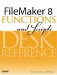# Choose()

Category: Logical

 Syntax: Choose ( test; result0 {; result1; result2...} )

Parameters:

testAn expression that returns a number greater than or equal to zero.

result(n)The value returned or the expression that is evaluated based on the result of the test. Parameters in curly braces { } are optional and may be repeated as needed, separated by a semicolon.

Data type returned: Text, Number, Date, Time, Timestamp, Container

Description:

Returns one of the result values according to the integer value of test. FileMaker evaluates test to obtain an index number, which is used to then select the corresponding ordinal result.

The Choose function is a 0-based list. Choose ( 1; "a"; "b"; "c" ) will return "b".

Any fractional value of test is ignored (as opposed to rounded) when obtaining the index number. Choose ( 1.9; "a"; "b"; "c" ) will return "b".

If the index value returned by test exceeds the number of results available, the Choose function will not return any resultThe field will be blank as opposed to having a "?" in it. There is no way to define a default value to use when the index value exceeds the number of results available.

Examples:

Function

Results

Choose ( DayOfWeek( Get ( CurrentDate ) ); ""; "Sun"; "Mon"; "Tue"; "Wed"; "Thu"; "Fri"; "Sat")

Returns a three-letter day name abbreviation for today's date.

Choose ( ( Month ( myDate )/ 3.1 ); "Q1"; "Q2"; "Q3"; "Q4")

Returns "Q1" for the instance where myDate contains "2/1/2005".

The following formula converts decimal values to fractional notation, rounded to the nearest eighth. Assume an input from a field (or parameter), myNumber.

```
```

[View full width]

Let ([ n = myNumber; int = int ( n ); decimal = mod ( n; 1 ); numberOfEighths = Round ( decimal/.125; 0 ); intDisplay = Case ( Abs ( int ) > 0; int & Case ( Abs ( decimal ) > 0; " - "; "" );"" ); fraction = Choose( numberOfEighths; Floor ( n ); intDisplay & "1/8"; intDisplay & "1/4"; intDisplay & "3/8"; intDisplay & "1/2"; intDisplay & "5/8"; intDisplay & "3/4"; intDisplay & "7/8"; Ceiling ( n ) ) // end choose ]; // end variables fraction )

If myNumber contained 3.45, this function would return 3 - 1/2.FileMaker 8 Functions and Scripts Desk Reference
ISBN: 0789735113
EAN: 2147483647
Year: 2004
Pages: 352